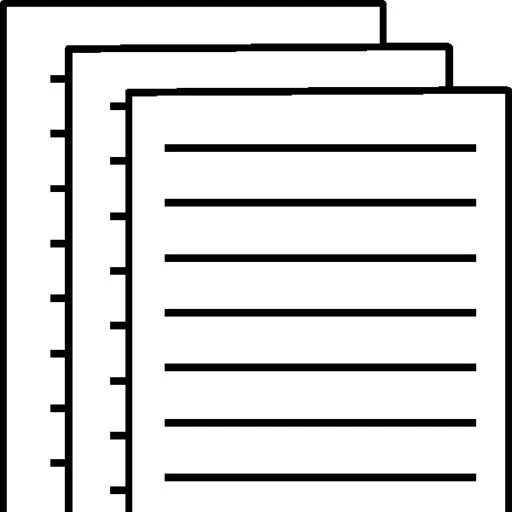## BBA 3301 Unit V Assignment

Instructions: Enter all answers directly in this worksheet. When you are finished, select Save As, and save this document using your last name and student ID as the file name. Upload the data sheet to Blackboard as a .doc, .docx or .rtf file when you are finished.

Question 1. (30 points total) Use this balance sheet and income statement from Carver Enterprises to complete parts a and b:

Common−Size Balance Sheet Cash and marketable securities Accounts receivable Inventories 2013 \$ 490 1.5 % 5,990 18.1 9,550 28.9 \$ 16,030 48.5 % 17,030 51.5 \$ 33,060 100.0 % \$ 7,220 21.8 % 6,800 20.6 \$ 14,020 42.4 % 7,010 21.2 \$ 21,030 63.6 % 12,030 36.4 \$ 33,060 100.0 %
Common−Size Income Statement Revenues Cost of goods sold Gross profit 2013 \$ 30,020 100.0 % (19,950) -66.5 \$ 10,070 33.5 % (7,960) -26.5 \$ 2,110 7 % (940) -3.1 \$ 1,170 3.9 % (425) -1.4 \$ 745 2.5 %

Question 2. (10 points total) Use this data table of Campbell Industries liabilities and owners’ equity to complete parts a and b.

• (15 points) Prepare a common size balance sheet for Carver Enterprises. Complete the common-size balance sheet: (Round to one decimal place.)
• (15 points) Prepare a common-size income statement for Carver Enterprises. Complete the common-size income statement: (Round to one decimal place.)
• (5 points) What percentage of the firm’s assets does the firm finance using debt (liabilities)? (Round to one decimal place.)
• (5 points) If Campbell were to purchase a new warehouse for \$1.3 million and finance it entirely with long-term debt, what would be the firm’s new debt ratio? (Round to one decimal place.)

Question 3. (10 points total) (Liquidity analysis) Airspot Motors, Inc. has \$2,433,200 in current assets and \$869,000 in current liabilities. The company’s managers want to increase the firm’s inventory, which will be financed using short-term debt. How much can the firm increase its inventory without its current ratio falling below 2.1 (assuming all other assets and current liabilities remain constant)? (Round to one decimal place.)

Question 4. (10 points total) (Efficiency analysis) Baryla Inc. manufactures high quality decorator lamps in a plant located in eastern Tennessee. Last year the firm had sales of \$93 million and a gross profit margin of 45 percent.

• (5 points) How much inventory can Baryla hold and still maintain an inventory turnover ratio of at least 6.3 times? (Round to one decimal place.)
• Question 5. (15 points total) (Profitability and capital structure analysis) In the year that just ended, Callaway Lighting had sales of \$5,470,000 and incurred cost of goods sold equal to \$4,460,000. The firm’s operating expenses were \$128,000 and its increase in retained earnings was \$42,000 for the year. There are currently 99,000 common stock shares outstanding and the firm pays a \$4.770 dividend per share. The firm has \$1,180,000 in interest-bearing debt on which it pays 7.7 percent interest.

• (5 points) Currently, some of Baryla’s inventory includes \$2.3 million of outdated and damaged goods that simply remain in inventory and are not salable. What inventory ratio must the good inventory maintain in order to achieve an overall turnover ratio of at least 6.3 (including the unsalable items)? (Round to one decimal place.)
• Income Statement Revenues \$ 5,470,000 4,460,000 \$ 1,010,000 128,000 \$ 882,000 90,860 \$ 791,140 276899 \$ 514,241
 The operating profit margin is The net income margin is 16.1 % 9.4 %
 The times interest earned ratio is 970.7 %

What does this tell you about Callaway’s ability to pay its interest expense? (Fill in the blank with the times interest earned ratio from above and select the best choice.)

• (5 points) Assuming the firm’s earnings are taxed at 35%, construct the firm’s income statement.
• (5 points) Calculate the firm’s operating profit margin and net profit margin. (Round to one decimal place.)
• (5 points) Compute the times interest earned ratio.

What is the fin’s return on equity? (Select the best choice.)

• Callaway’s operating income can fall as much as ______ times the interest expense and the company would still be able to service its debt.
• The firm’s return on equity is the same as the net profit margin, 9.4%.
• The firm’s return on equity is the sum of the operating profit margin and the net profit margin, 25.5%.
• There is not enough information to answer this question.
• The firm’s return on equity is the same as the operating profit margin, 16.1%.

Question 6. (5 points total) (Market value analysis) Lei Materials’ balance sheet lists total assets of \$1.16 billion, \$132 million in current liabilities, \$415 million in long-term debt, \$613 million in common equity, and 58 million shares of common stock. If Lei’s current stock price is \$52.08, what is the firm’s market-to-book ratio? (Round to one decimal place.)

Question 7. (5 points total) (DuPont analysis) Bryley, Inc. earned a net profit margin of 5.1 percent last year and had an equity multiplier of 3.49. If its total assets are \$109 million and its sales are \$157 million, what is the firm’s return on equity? (Round to one decimal place.)

Question 8. (15 points total) (Calculating financial ratios) Use the balance sheet and income statement for the J. P. Robard Mfg. Company to calculate the following ratios:

 Current ratio (Round to two decimal places.) Times interest earned (Round to two decimal places.) 1.65 4.55 times 3.67 times 1.03 20.7 % 21.2 % 50.5 % 87.7 days 1.78 20.1 %

To view and download a complete answer, scroll down to the bottom to pay